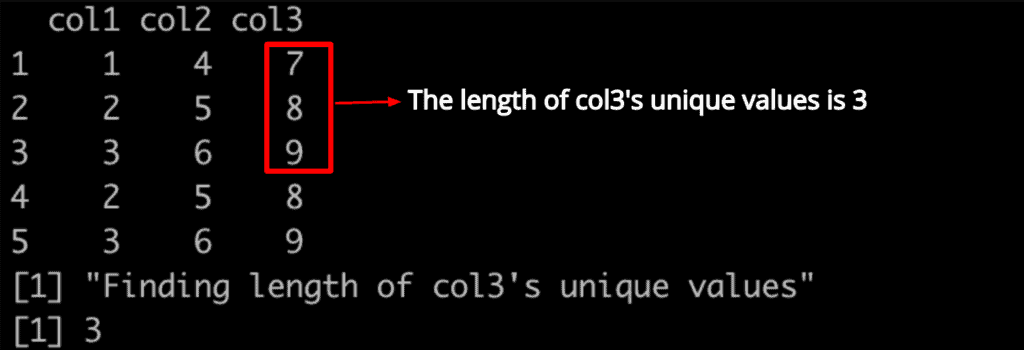# How to Use unique() Function in R

The unique() function in R is used to eliminate or remove the duplicate values or the rows present in the data frame, matrix, and vector.

## Syntax

``unique(data)``

## Parameters

data: It is a vector, data frame, array, or NULL.

## Return Value

1. If the input data is a Vector, it returns an object of the same type of data input but with only one copy of each duplicated element.
2. If the input data is a data frame, it returns a data frame with the same columns but possibly fewer rows.
3. If the input data is a matrix or array, it is subsetted by [, drop = FALSE], so dimensions and dimnames are copied appropriately. The result always has the same dimensions as the input data.

## Visual Representation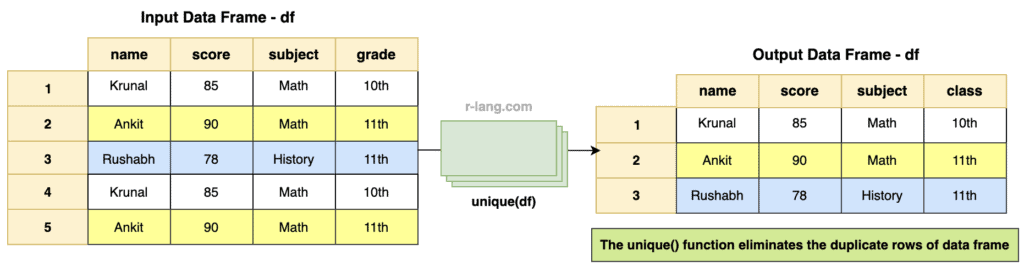The diagram tells us that applying the unique() function on the data frame will remove the duplicate rows from the data frame and return the data frame with unique rows.

## Example 1: Finding unique values in a vector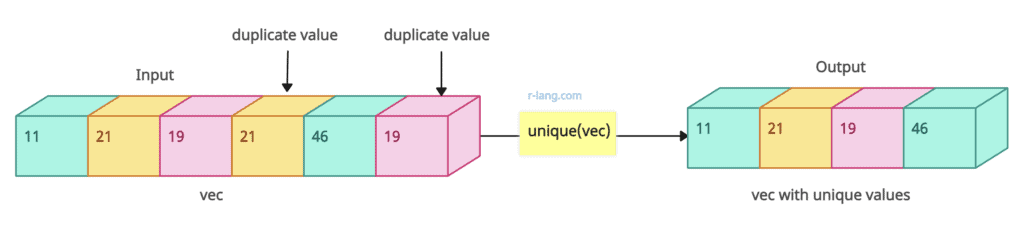The diagram tells us that the unique() function removes the duplicate values from the vector and returns a vector with unique values.

``````vec <- c(11, 19, 11, 21, 46, 19)

unique(vec, incomparables = FALSE)``````

Output

`` 11 21 19 46``

## Example 2: Finding unique values from a matrix

If you have a matrix with duplicate values, the unique() function will help you remove the duplicate rows and return the matrix with unique ones.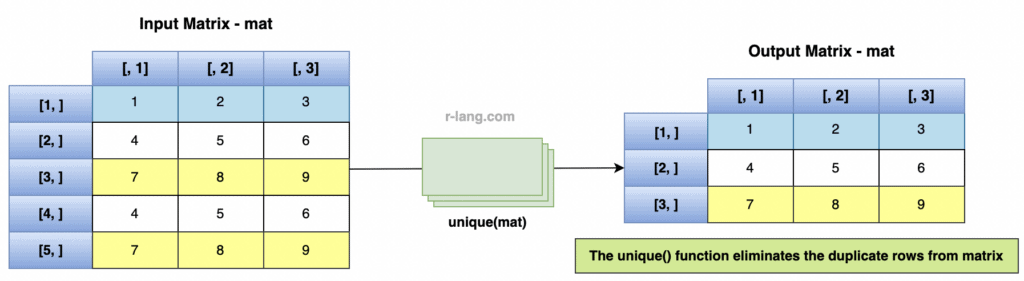``````rv <- c(1, 2, 3, 4, 5, 6, 7, 8, 9, 4, 5, 6, 7, 8, 9)
mtrx <- matrix(rv, nrow = 5, ncol = 3, byrow = TRUE)
print(mtrx)

cat("Unique values from matrix", "\n")
unique(mtrx)
``````

Output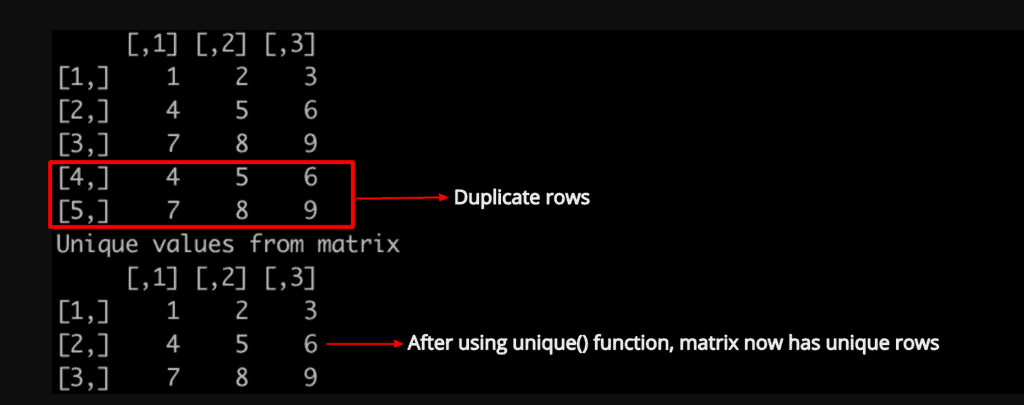If fromLast=TRUE, the duplicated row will be considered from the last backward.

``````mtrx <- matrix(rep(1:12, length.out = 18), nrow = 6, ncol = 3, byrow = T)
mtrx
cat("After removing duplicates", "\n")
unique(mtrx, fromLast=T)``````

Output

``````     [,1] [,2] [,3]
[1,]  1    2    3
[2,]  4    5    6
[3,]  7    8    9
[4,] 10   11   12
[5,]  1    2    3
[6,]  4    5    6

Unique values from matrix

[,1] [,2] [,3]
[1,]   7   8    9
[2,]  10   11   12
[3,]   1   2    3
[4,]   4   5    6``````

## Example 3: Finding unique rows in a data frame

To find the unique rows of a data frame, use the “unique()” method and pass the data frame as an argument, and the method returns unique rows.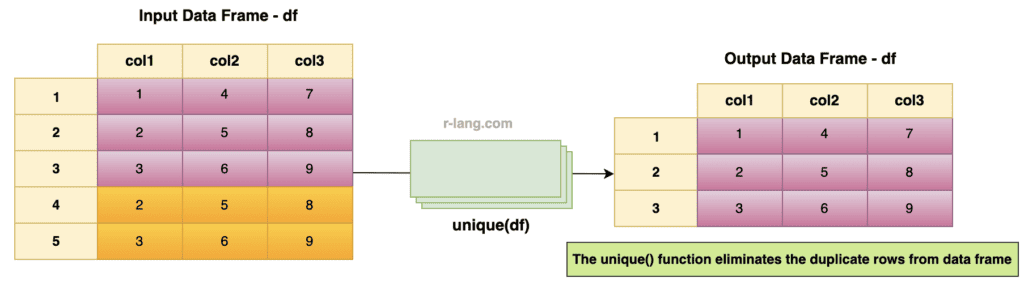``````df <- data.frame(
col1 = c(1, 2, 3, 2, 3),
col2 = c(4, 5, 6, 5, 6),
col3 = c(7, 8, 9, 8, 9)
)
df
print("Applying unique() function to get unique values")
unique(df)
``````

Output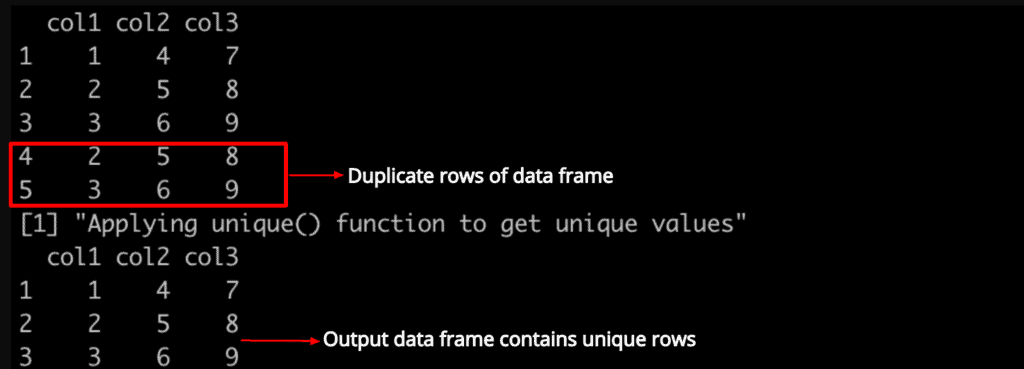## Example 4: Finding unique values of specific columns in the data frame

``````df <- data.frame(
col1 = c(1, 2, 3, 2, 3),
col2 = c(4, 5, 6, 5, 6),
col3 = c(7, 8, 9, 8, 9)
)

df

print("Applying unique() function on col3 to get unique values")
unique(df\$col3)
``````

Output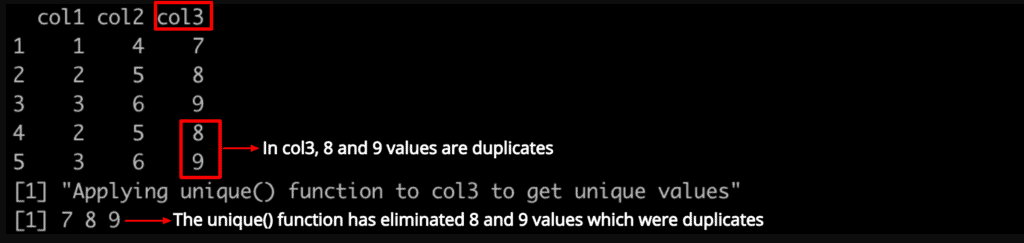## Example 5: Finding the length of unique values of a specific column

To find the length of unique values of a specific data frame column or vector, you can use the “length()” function.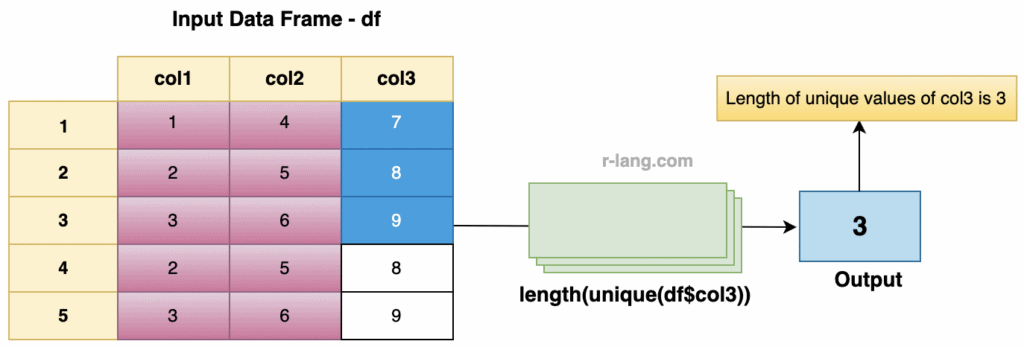``````df <- data.frame(
col1 = c(1, 2, 3, 2, 3),
col2 = c(4, 5, 6, 5, 6),
col3 = c(7, 8, 9, 8, 9)
)

df

print("Finding length of col3's unique values")

col3_unique_val_length <- length(unique(df\$col3))
col3_unique_val_length
``````

Output